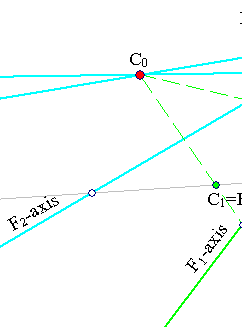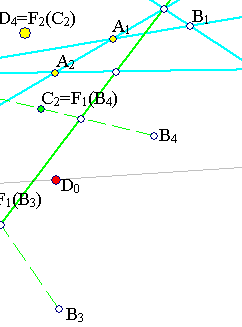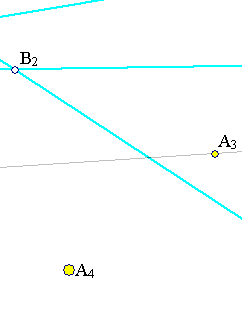##Projectivity resolution in Perspectivities

Every projectivity can be represented as the product of at most four perspectivities.Given the projectivity F, select four points {Ai} in general position and consider the image points {Bi}, which are also in general position. By composing F with two appropriate harmonic perspectivities F1, F2, we can arive at a perspectivity F' = F2*F1*F, leaving fixed three of the points {Ai}, say for i=1,2,3, and mapping A4 to some other point D4. F' is then easily seen to be a product of elations, F' = E2*E1 = F2*F1*F . Thus, implying F = F2-1*F1-1*E2*E1, which is a product of four perspectivities.
The appropriate F1 is the harmonic perspectivity interchanging A1 with B1 and A2 with B2. Its axis and center (C0) are determined by the complete quadrilateral A1B1B2A2.
Applying F1 to B3, B4 we find C1= F1(B3) and C2=F2(B4).
The appropriate F2 is the harmonic perspectivity with axis the line A1A2 and center D0, such that it interchanges C1 and A3. It is easily verifyied that F' = F2*F1*F behaves as it should i.e. leaves {Ai, i=1,2,3} fixed and maps A4 to D4. To see that F' is a product of elations, consider the standard model of projective plane in which points are identified with lines [x1,x2,x3]. Take a basis whose vectors span {Ai, i=1,2,3} and calculate the matrix representing F' (see Perspectivity.html ). It is easily seen, that in this base F' is represented with the diagonal matrix M = diag(d1/a1,d2/a2,d3/a3), where [a1,a2,a3] and [d1,d2,d3] represent points A4 and D4 respectively. Since the projectivity is defined by the matrix modulo a multiplicative constant, this can be reduced to a diagonal matrix of the form diag(a,b,1), which is a product of the two diagonal matrices diag(a,1,1) and diag(1,b,1), representing the elations.
All the elementary matrices represent perspectivities (this subject is examined in the file Elation.html ). This could be used to give an alternative proof of the above property, based on the decomposition of an invertible matrix in elementary ones.
From the above arguments follows, more precisely, that every projectivity is the product of two harmonic perspectivities and two elations. On the other hand, as exposed in ElationDecomposition.html , every elation is a product of two harmonic perspectivities. Thus, every projectivity is a product of harmonic perspectivities, the product involving at most six factors.